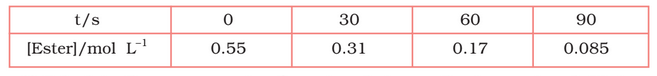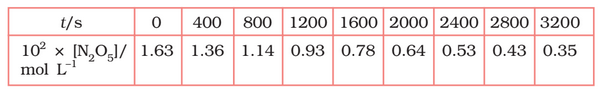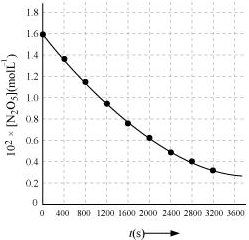# NCERT Solutions for Class 12 Chemistry Chapter 4 Chemical kinetics

NCERT solutions for class 12 chemistry chapter 4 Chemical Kinetics - Chemical kinetics deals with the average and instantaneous rate of reaction, factors affecting these and the mechanism of the reaction. Chemical kinetics helps students to understand how chemical reactions occur. In NCERT solutions for class 12 chemistry chapter 4 Chemical Kinetics, there are questions and solutions of some important topics like average and instantaneous rate of a reaction, factors affecting the rate of reaction, the integrated rate equations for the zero and first-order reactions, etc. This chapter also tells you when is a chemical reaction feasible and how can we calculate the speed of reactions.

This chapter holds 5 marks in the class 12 CBSE board exam of chemistry. In this chapter, there are 30 questions in the exercise and 9 questions which are related to topics studied. To clear doubts of students, the CBSE NCERT solutions for class 12 chemistry chapter 4 Chemical Kinetics are prepared in a comprehensive manner by subject experts. This chapter is vital for both CBSE Board exam as well as for competitive exams like JEE Mains, VITEEE, BITSAT, etc. so, students must pay special attention to concepts of this chapter. The NCERT solutions provided here are completely free and you can also download them for offline use also if you want to prepare or any other subject or any other class.

Intext Questions

Exercise Questions

Rate of reaction- It is defined as the rate of change in concentration of reactant or product. Unit of rate is .

Reactants, R  Products, P

nA+mB  pC+qD

## Topics and Sub-topics of NCERT Class 12 Chemistry Chapter 4 Chemical Kinetics-

4.1Rate of a Chemical Reaction

4.2Factors Influencing Rate of a Reaction

4.3Integrated Rate Equations

4.4Temperature Dependence of the Rate of a Reaction

4.5 Collision Theory of Chemical Reactions

## NCERT solutions for class 12 chemistry chapter 4 Chemical Kinetics -

Solutions to In Text Questions Ex 4.1  to 4.9

We know that,

The average rate of reaction =

=

=

=

In seconds we need to divide it by 60. So,

=

= 6.67

According to the formula of an average rate

=             (final concentration - initial conc.)/time interval

=

=

=

=

Order of reaction = Sum of power of concentration of the reactant in the rate law expressions

So, here the power of A = 0.5

and power of B = 2

order of reaction  = 2+0.5 =2.5

The order of a reaction means the sum of the power of concentration of the reactant in rate law expression.

So rate law expression for the second-order reaction is  here R = rate

if the concentration is increased to 3 times means

new rate law expression =  = =  9R

the rate of formation of Y becomes 9 times faster than before

Given data,

initial conc. = 5g

final conc. = 3g

rate const. for first-order =

We know that for the first-order reaction,

[log(5/3)= 0.2219]

= 444.38 sec (approx)

We know that t(half ) for the first-order reaction is

and we have given the value of half time

thus,

= 0.01155 /min

OR = 1.1925

Alternative method

we can also solve this problem by using the first-order reaction equation.

put

The rate constant of the reaction is nearly doubled on rising in 10-degree temperature.

Arrhenius equation depicts the relation between temperature and rates constant.

A= Arrhenius factor

Ea = Activation energy

R = gas constant

T = temperature

Given data

(initial temperature) = 298K and  (final temperature)= 308K

And we know that rate of reaction is nearly doubled when temperature rise 10-degree

So,  and R = 8.314 J/mol/K

now,

On putting the value of given data we get,

Activation energy () =

=52.9 KJ/mol(approx)

We have

Activation energy = 209.5KJ/mol

temperature= 581K

R = 8.314J/mol/K

Now,  the fraction of molecules of reactants having energy equal to or greater than activation energy is given as

taking log both sides we get

= 18.832

x = antilog(18.832)

= 1.471

## NCERT Solutions for class 12 chemistry chapter 4

Given pieces of information

Rate =

so the order of the reaction is 2

The dimension of k =

(ii)

Given rate =

therefore the order of the reaction is 2

Dimension of k =

Given

therefore the order of the reaction is 3/2

and the dimension of k

so the order of the reaction is 1

and the dimension of k =

The initial rate of reaction =

substitute the given values of [A], [B] and k,

rate  =

=8

When [A] is reduced from 0.1 mol/L to 0.06 mol/L

So, conc. of A reacted = 0.1-0.06 = 0.04 mol/L

and conc. of B reacted = 1/2(0.04) = 0.02mol/L

conc. of B left = (0.2-0.02) = 0.18 mol/L

Now,  the rate of the reaction is (R) =

=

The decomposition of  on the platinum surface reaction

therefore,

Rate =

For zero order reaction rate = k

therefore,

So

and the rate of production of dihydrogen  = 3(2.5

= 7.5

The following factors that affect the rate of reaction-

• the concentration of  reactants

• temperature, and

• presence of catalyst

Let assume the concentration of reactant be x

So, rate of reaction,

Now, if the concentration of reactant is doubled then  . So the rate of reaction would  be

Hence we can say that the rate of reaction increased by 4 times.

Let assume the concentration of reactant be x

So, rate of reaction, R =

Now, if the concentration of reactant is doubled then  . So the rate of reaction would  be

Hence we can say that the rate of reaction reduced to 1/4 times.

The rate constant is nearly double when there is a 10-degree rise in temperature in a chemical reaction.

effect of temperature on rate constant be represented quantitatively by Arrhenius equation,

where k is rate constant

A is Arrhenius factor

R is gas  constant

T is temperature and

is the activation energy(i) Calculate the average rate of reaction between the time interval 30 to 60 seconds.

The average rate of reaction between the time 30 s to 60 s is expressed as-

Question 4.9(i)     A reaction is first order in A and second order in B.

(i)Write the differential rate equation.

the reaction is first order in A and second order in B. it means the power of A is one and power of B is 2

The differential rate equation will be-

Question 4.9(ii)    A reaction is first order in A and second order in B.

(ii) How is the rate affected on increasing the concentration of B three times?

If the concentration of [B] is increased by 3 times, then

Therefore, the rate of reaction will increase 9 times.

Question 4.9(iii)     A reaction is first order in A and second order in B.

(iii) How is the rate affected when the concentrations of both A and B are doubled?

If the concentration of [A] and[B] is increased by 2 times, then

Therefore, the rate of reaction will increase 8 times.we know that
rate law () =
As per data

these are the equation 1, 2 and 3 respectively

Now, divide eq.1 by equation2, we get

from here we calculate that y = 0

Again, divide eq. 2 by Eq. 3, we get
Since y =0 also substitute the value of y
So,
=
=

taking log both side we get,

= 1.496
= approx 1.5
Hence the order of reaction w.r.t A is 1.5 and w.r.t B is 0(zero)The given reaction is first order wrt A and zero order in wrt B. So, the rate of reaction can be expressed as;

Rate = k[A]

from exp 1,

k = 0.2 per min.

from experiment 2nd,

[A]

from experiment 3rd,

from the experiment 4th,

from here [A] = 0.1 mol/L

We know that,
half-life () for first-order reaction =
=

the half-life for the first-order reaction is expressed as ;

=  0.693/2
= 0.35 min (approx)

The half-life for the first-order reaction is
= 0.693/4
=  0.173 year (approximately)

Given ,
half-life of radioactive decay = 5730 years
So,
per year

we know that, for first-order reaction,

= 1845 years (approximately)

Thus, the age of the sample is 1845 years

Question 4.15 (1)    The experimental data for decomposition of

in gas phase at 318K are given below:Plot against t.